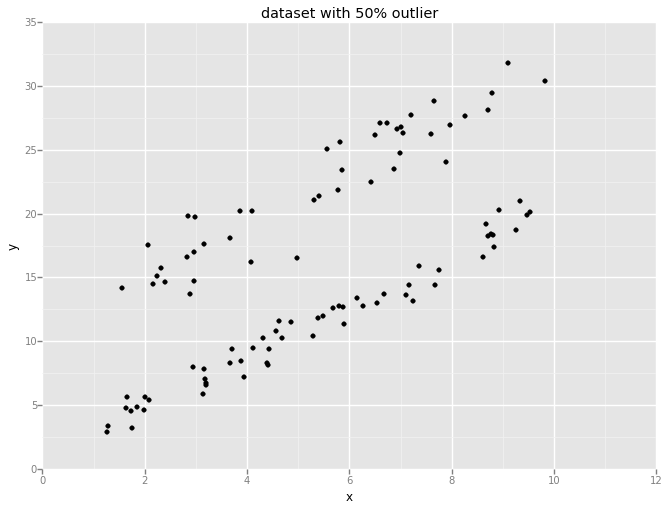Using RANSAC for Robust Regression

An Outlier is defined as a point (or set of points) in the dataset that do not follow the dominant pattern of the data. Due to wide variety of outlier sources, from variability in the measurement to high power noise in data collection, it is almost impossible to find a real world dataset without outliers. Hence it is absolutely vital to make sure that the data modeling is robust to the outlier. A robust machine learning algorithm is defined as the one that provides high performance even in the existence of outliers. Without robust learning the insight to the data is biased and does not have the correct model.

In this post, I would like to touch the surface of outlier detection and removal by introducing Random Sample Consensus. RANSAC is a a non-deterministic iterative algorithm that estimates the parameter of a (supervised) machine learning algorithm from a dataset that contains outliers. For that, RANSAC divides the points in the dataset into two subsets: 1- outlier 2- inlier. Then it uses the inliers to create the ML model.

Generally speaking, RANSAC starts by selecting a subset of points as hypothetical inliers. The size of this subset is selected big enough to fit the ML model. For example for linear regression we need at least n+1 points where n is the dimension of the features. After fitting the model to the hypothetical inliers, RANSAC checks which elements in the original dataset are consistent with the model instantiated with the estimated parameters and, if it is the case, it updates the current subset. The RANSAC algorithm iteratively repeats until the inlier subset is large enough (large enough is an input to the algorithm) or reaching to the end of the iteration.

RANSAC algorithm is designed based on two fundamental assumptions.
1. There are enough inliers points to agree on a good model
2. The outliers will not vote consistently for any single model. This is vital because otherwise the outliers will consistency create their own model and the iteration will fall into local optimum.
Example:
(codes in Python using scikit-learn module)
Let study the performance of RANSAC in a linear regression problem. Let create a simple 1D dataset that has 10% outliers as depicted in the following plot:A dataset with 10% outlier for linear regression y=2x+1.

Clearly, in above plot x has linear relation with y (y=2x+1) with small points that do not fall into this pattern. Let first fit a linear model as follows:
from sklearn import linear_model
clt = linear_model.LinearRegression()
clt.fit(feature, y)
yhat = clt.predict(feature)
print clt.coef_
print clt.intercept_
--
[ 2.17529381]
3.34794649807
The following plot shows the linear regression result. As one can see, outliers have influenced the regression model.Ordinary linear regression. The model is biased toward the outliers.
Now, let use RANSAC to create a robust linear regression.
clt_ransac = linear_model.RANSACRegressor(linear_model.LinearRegression())
clt_ransac.fit(feature, y)
yhat_ransac = clt_ransac.predict(feature)
The last line, creates a boolean array to describe whether the corresponding point in feature vector is an outlier or not. If we look at the linear regression coefficients we can see that it is closer to the actual:
print clt_ransac.estimator_.coef_
print clt_ransac.estimator_.intercept_
--
[[ 1.98733376]]
[ 1.57728374]
The following plot shows the result of linear regression and outlier points that has marked and removed by RANSAC for fitting the linear regression.RANSAC divides the dataset into inliers and outliers. The inliers is used to fit the linear regression.

Large Outlier Population
As the last example, let increase the percentage of outlier points to 50% and use RANSAC and linear regression.A dataset with 50% outlier.RANSAC is strongly robust to the size of the outliers population as long as the inliers consistently vote for single model.

The slope and intercept of the model is given as below:
[[ 1.90050698]]
[ 1.47968426]

Favorite Quotes

"I have never thought of writing for reputation and honor. What I have in my heart must out; that is the reason why I compose." --Beethoven

"All models are wrong, but some are useful." --George Box